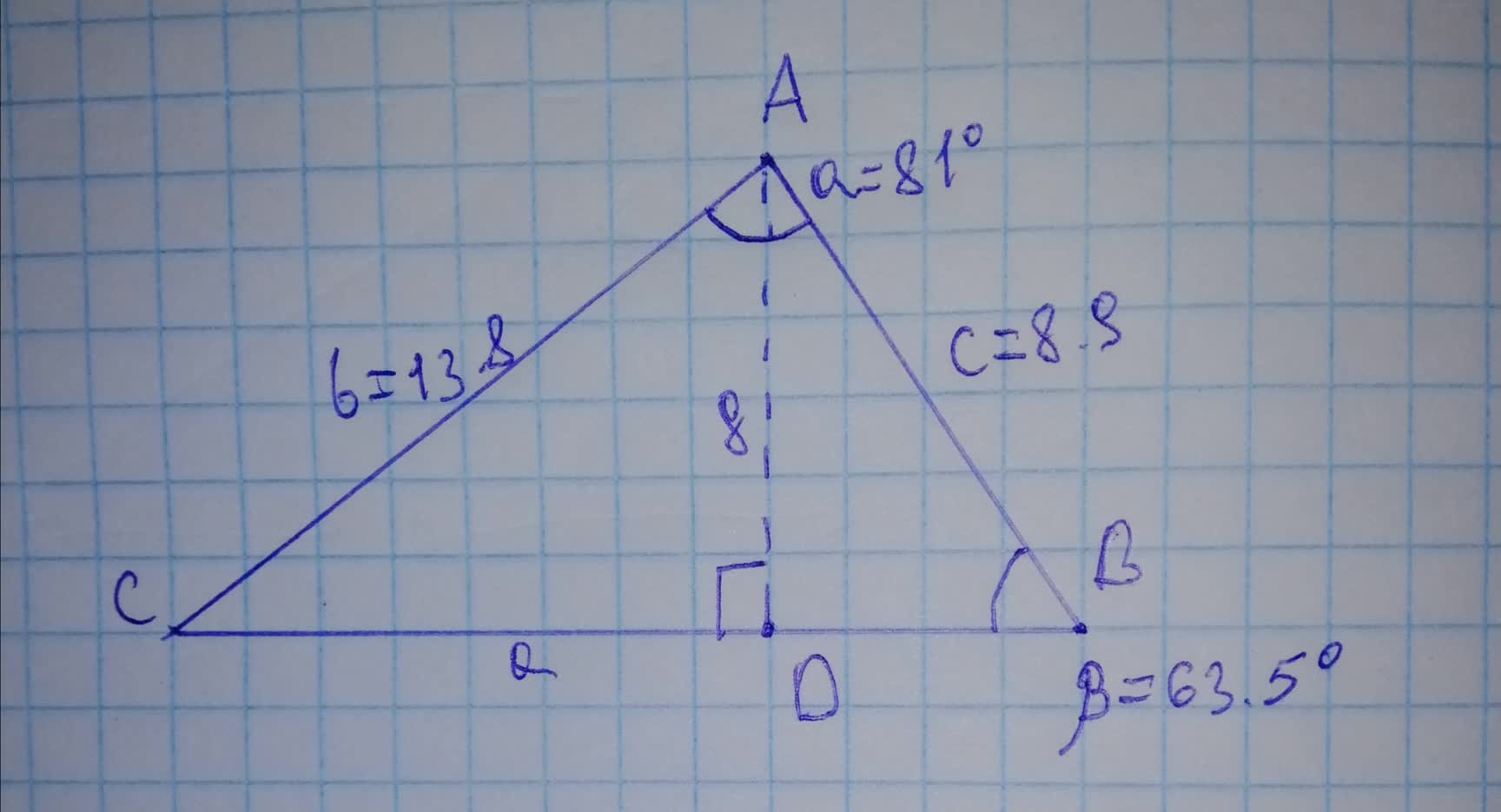# Find the height of the triangle g to the nearesttapetivk 2021-11-23 Answered
Find the height of the triangle g to the nearest tenth.• Questions are typically answered in as fast as 30 minutes

### Plainmath recommends

• Get a detailed answer even on the hardest topics.
• Ask an expert for a step-by-step guidance to learn to do it yourself.May Dunn
Step 1
From the given data,
Consider the triangle ABD
Opposite side $$\displaystyle={g}$$
Hypotenuse $$\displaystyle{\left({c}\right)}={8.9}$$
$$\displaystyle\beta={63.5}^{{\circ}}$$
Step 2
We know that,
$$\displaystyle{{\sin{{63.5}}}^{{\circ}}=}{\frac{{{g}}}{{{8.9}}}}$$
$$\displaystyle{0.894934361}={\frac{{{g}}}{{{8.9}}}}$$
$$\displaystyle{g}={8.9}\times{0.894934361}$$
Therefore,
$$\displaystyle{g}={7.964915818}$$ units.
Rounding off to the nearest tenth we have,
$$\displaystyle{g}={8.0}$$ units is the required answer.